## Oblate Spheroidal Wave Function

The wave equation in Oblate Spheroidal Coordinates is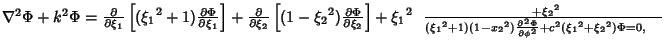(1)
where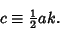(2)

Substitute in a trial solution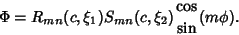(3)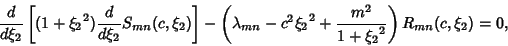(4)

and the angular differential equation is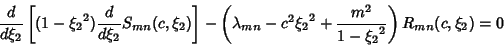(5)

(Abramowitz and Stegun 1972, pp. 753-755).

Abramowitz, M. and Stegun, C. A. (Eds.). Spheroidal Wave Functions.'' Ch. 21 in Handbook of Mathematical Functions with Formulas, Graphs, and Mathematical Tables, 9th printing. New York: Dover, pp. 751-759, 1972.